Commercial slide rules

Introduction

A number of slide rules were produced to aid commercial calculations.These instructions are based on six rules:

Blundell 808 Accountant (UK) - for which I have the instructions.
Faber Castell Novo Mentor 52/81 (Germany) - for which I have the instructions.
Faber Castell  Super business 1/28 (Germany) - for which I don't have the instructions.
Pickett 510 (USA) - for which I have the instructions.
Nestler 7/52 (Germany) - for which I don't have have the instructions
Graphoplex 645 (France) - for which I have the instructions

Fortunately there is a degree of overlap between the functionality of the rules so from the information available it has been possible to work out how the rules without instructions can be used.

The special scales are used for three main purposes:

1. To calculate profit and discount. Others names for these calculations are profit and mark-up. They relate to the fact that if you buy something for £10 and sell it for £15 this can be considered as a mark-up of 50% on the purchase price or a profit of 331/3 % on the retail price. If something is normally sold at £15 but is sold at discounted price of £10 the discount is also 331/3 %. On some rules there are special scales for this and on others special markings on "standard" scales. One interesting feature is the number of different ways that are used for thus particular calculation.
2. Calculations of interest. All the above rules allow for simple interest and some allow for compound interest. In the case of compound interest the scales used are log-log scales but usually named differently to standard rules.
3. Conversion of pre-decimal British currency to decimal equivalents

One, at first, unusual feature of many commercial rules is that the CF and DF scales are often folded at 360, rather 365 the number of days in the year or the more normal p. This apparently reflects normal commercial practice of the use of 1/360 of the annual rate of interest for periods of less than a year.

Many of the rules also have scales for the conversion of the previous British currency units into decimal equivalents. This was done even before the currency was decimalised in 1971. Before that date the basic unit was the pound sterling, as it is now. The pound was divided in 20 shillings, each of which had 12 pence. The penny was divided in half pennies (written ha'pence) and farthings, one quarter of a penny but largely obsolete at the time of decimalisation. Amounts were generally written as £2 7s 6d, the £sd being derived from the Latin libra, solidus and denarius. Amounts less than a pound were often written 7/6, for seven shillings and sixpence or -/31/2 for amounts less than a shilling.

Since many of the rules have similar scales but with different names, I have not attempted to describe each example of typical calculations on each slide rule. At the end of this section I give a tables of scale equivalence.

The rules

Blundell 808 Accountant

This rule has a full range of scales for profit (called Uplift), discount (not shown) and interest (simple and compound). Two uncommon features on this rule are the way the DF and CF scales are folded, at .00274 (i.e. 1/365), and the scale on the back of the rule for finding the number of days between any two dates.

 Detail - front left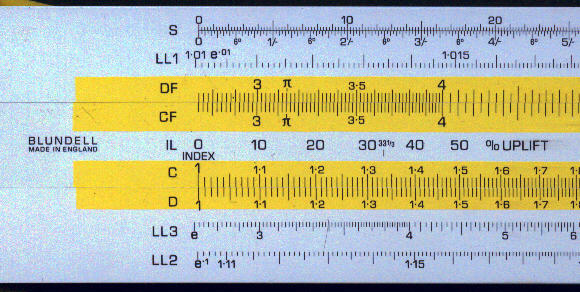Detail - date scales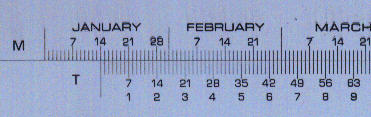Faber Castell Novo Mentor 52/81

This rule has what my be considered a rather inappropriate set of scales for business use. It includes trig scales, with a sine scale on both the slide and the stock and which would rarely be needed for commercial calculations, but does not have any log-log scales for compound interest calculations. The profit and discount scales are on the DF scale centred on the 1 mark.

The CF and DF scales are folded at p , as is normal on general purpose slide rules but not particularly relevant for commercial rules, however the cursor has special lines to enable multiplication by 360 or 365.

The front view shows the main cursor mark set on 1 on the scale and to the top right at 360 on the DF scale. The slide has also been position with the main cursor line over the 360 on the central (CI) scale and small cursor line lies over the 365. The cursor also hair lines for conversion between horse-power (HP) and kilowatts (kW). This image also shows the scale markings: K - capital and Z - interest.

The centre image shows the profit and discount scales. These are centred on the 1 of the DF scale.

The third image shows the trig scales on the back of the rule including the duplicate sine scales.

 Front view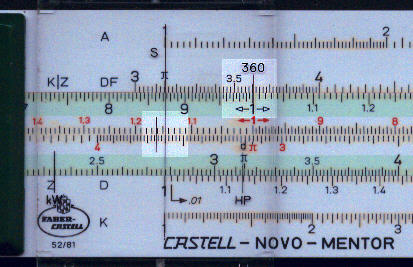Profit and discount scales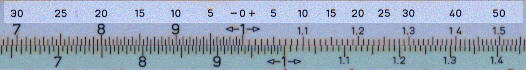Back view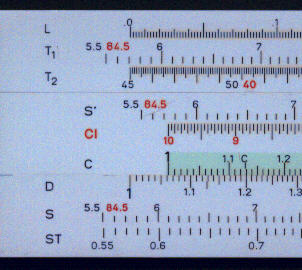This rule has scales for compound interest and is folded at 360. It does not have scales specially arranged for profit and discount.

The cursor has a similar mark to that on the previous rule for use with 365 days. The other two cursor lines correspond to p/4.

The scales on the front of the rule have the standard labels at the left hand end (DF, D etc) but at the right hand end have markings appropriate for commercial use. The are also gauge points for numbers, such as 12 or 144, frequently used in commercial calculations.

On the lower edge of the rule there is a scale of decimal equivalent of pounds, shillings and pence. In addition to this scale there is also a table of decimal equivalents of British currency.

The reverse of the slide has log-log scales for compound interest. This has percentages indicated to facilitate interpretation of the scales (the value for 1% highlighted below).

 Front view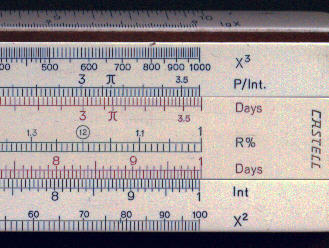Conversion of British currency to decimal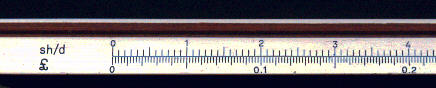Slide reversed.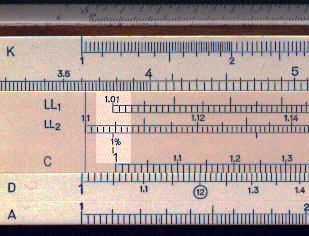Pickett 510 Business and Finance Rule

This rule has full range of scales for commercial use including log-log scales (labelled S1 to S3). There are two special scales for profit and discount.

The rule has two scales for "cost mark-up" labelled C and for retail mark-up" labelled R. These are shown highlighted in the two front views. It also has a set of log-log scales, labelled S1 to S3, these start with lower values than is often the case to allow lower rates of interest.

 Front view - left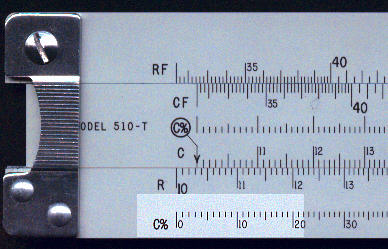Front view - right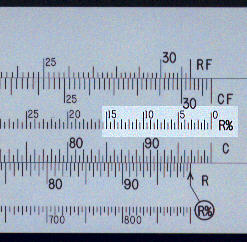Back view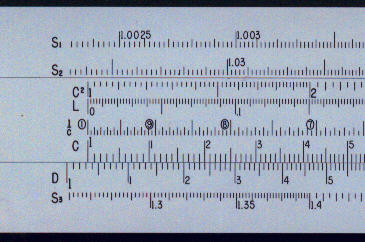Nestler 7/52

This is the most basic of the rules considered here. Apart from the fact that the rule is folded at 360 and the scales have labelling (with German initial letters) suitable for business there is little to distinguish it from other rules. The labelling of the scales is E (Einkauf - Purchase), V (Verkauf - Sale), T (time), Z (interest) and K (capital).

The cursor lines are set to multiply by 12, a factor often needed in commercial calculations.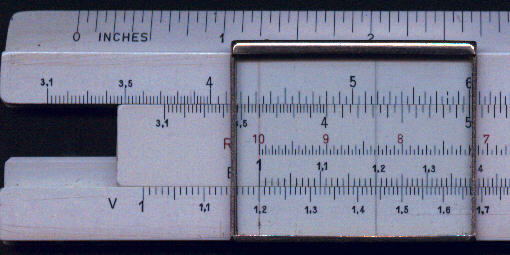Graphoplex 645 - Système Commercial

This rule also has a full set of commercial scales. It also has conversion scales for British currency and a set of factors for converting imperial/US/European units.

The first image shows the basic scales, which are labelled as on a standard rule, and some of the conversion factors. The CF and DF scales are folded at 360. As  on other rules, the profit/discount scales are centred on the DF scale. The log-log scales are on the back of the slide which has to be turned to enable it to be used.

The cursor has only a single line.

 Front view - left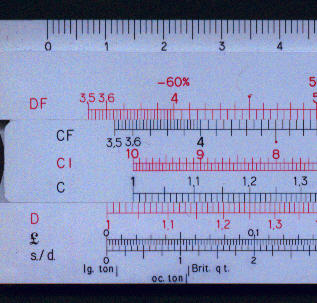Front view - right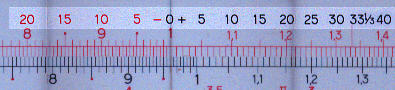Back view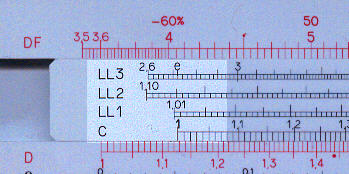Mark-up and profit calculations

The manual for the Pickett 510 considers 6 types of profit and mark-up calculations:
Cost and retail known: find the percent mark-up.
Cost and retail mark-up known: find the retail price.
Cost and cost mark-up known: Find the retail price
Retail price and retail mark-up known: find cost price.
Retail price and cost mark-up known: find the cost price
Cost mark-up percent know: find the retail mark-up percent.

In reality, it is only necessary to understand the basic concept, that the percent difference between the cost price and the selling price is different depending on whether it is calculated relative to the cost (or purchase price) price or to the sale price (or retail price). We will therefore consider a single very simple example. An item is purchased for £12 pounds and sold for £15. What is the percentage mark-up expressed relative to the purchase price and what is the percentage profit expressed relative to the selling price.

Let us consider the calculation first on the Pickett and Blundell rules, since their scales are equivalent. The first image, below, shows the Pickett and as can be seen by aligning the cost price on the C scale against the sale price on the R (or D) scale the answer (25%) to the first part of the question is found on the C% scale, or the %Uplift scale on the Blundell.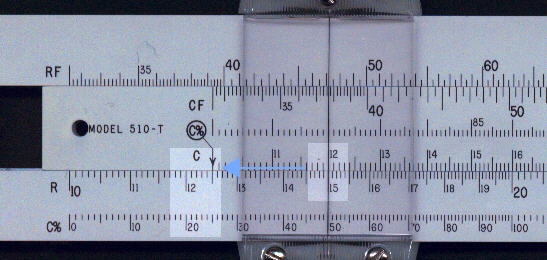For the answer to the second part of the question we have to look at the other end of the rule. In this case we show the Blundell and can see the answer (20%) opposite the 1 on the %Discount scale (or the R% scale on the Pickett).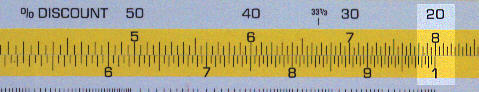These two rules described above are the only ones which allow you to get the answer to both parts of the problem without having to re-set the scale. If we consider next the two rules, the Graphoplex and Faber Castell Novo-Mentor, which have a similar scale arrangement we will see the two parts of the answer require separate calculations. The first image shows the calculation of the percentage relative to the cost price on the Faber Castell. By aligning the sale price on the DF scale with the cost price on the CF scale, we get the answer (25%) using the specially marked percentages on the  CF scale opposite the 1 on the D scale.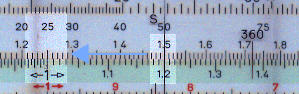For the second calculation we use the Graphoplex. In this case the order of calculation is reversed with the cost price on the upper DF scale and the sale price on the lower CF scale. The answer (20%) is still found on the CF scale opposite the 1 on the DF scale.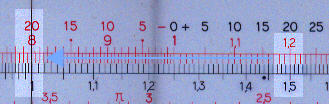Simple interest

Let us assume you arrange a bridging loan of £7 500 from 1 March to 21 June at an agreed rate of interest of 8.0% per annum.  How much will you have to repay at the end of the period.

Although the data scale on the Blundell is not generally available let us use it to calculate the loan period.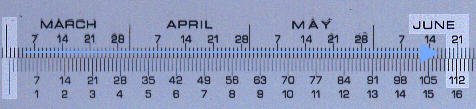Set the 1 on the number of days scale against the 1 March and read the duration (113 days) against 21 June on the number of days scale.

The basic formula is:
Repayment = Principal +  Interest
and
Interest = Rate * Duration / 100 = Principal * (8.0/360) * (113/100)

It is necessary to divide the 8.0% annual rate by 360 to get the daily rate. The example shown uses the Nestler which the CF and DF scales folded at 360. Align the 1 on the CF scale against the number of days on the DF scale. Align the cursor with the interest on the CF scale. Align 7 500 on the CI scale with the cursor and read the answer (£188) on the D scale against the 1 on the C scale.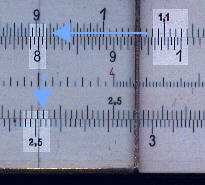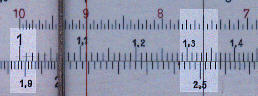Compound Investment

You have a choice of investing £4 500 in two bonds. One pays and interest of 5% for 15 years. The other pays 4% but has a terminal bonus of 10%. Which is the better investment.

The formula for compound interest is:
Final value = Initial value * (1 + interest/100)Period

This calculation has to be done is two stages. First calculate (1 + interest/100)Period . To do this we use the Pickett.

Set align the 1 of the C scale against 1 + interest/100 (1.05) on the S2 scale. Move the cursor to 15 years on the C scale and read the answer (2.08) on the S3 scale. Next multiply 2.08 by 4 500 (calculation not illustrated) and the answer is £9 360.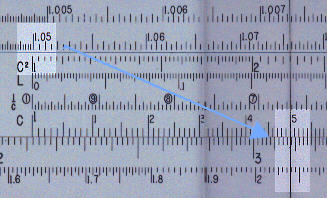Now let us consider the second alternative, this time using the Faber Castell 1/28 which has the interest scale on the slide. In this case the slide has to removed and replaced with the LL scales to the front. Align 1.04 (1 + interest/100) on the LL1 scale against the 1 on the D scale. Move the cursor to 15 on the D scale. Read the answer (1.80) on the LL2 scale. Now it necessary to multiply the investment (£4 500) by the multiplier just calculated (1.80) and then by the terminal bonus (1 + 10/100). The answer (this part of the calculation not illustrated) is £8920. The first investment is therefore to be preferred.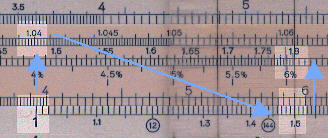Scale equivalence

Below we give the equivalence between the scales on the different rules. The column headed "description" uses the standard designation (e.g. D, CF etc) if there is one otherwise brief description of the scale's purpose.

 Description Blundell 808 FC 52/81 FC 1/28 Pickett 510 Nestler 7/52 Graphoplex 645 C scale C T, C C, days C E, T C D scale D Z, D D, Int R V, Z D CF CF: fold 1/360 T, CF: fold p CF, Days CF: fold p T, fold 360 CF DF DF: fold 1/360 K|Z,DF: fold p DF, P/Int: fold 360 RF: fold p KZ, fold 360 DF CI p% CI, R% R, % CI LL1 LL1: 1.01 to 1.14 LL1: 1.01 to 1.14 S1: 1.002 to 1.023 LL1: 1.01 to 1.11 LL2 LL2: 1.14 to 2.72 LL2: 1.14 to 2.72 S2: 1.023 to 1.026 LL2: 1.10 to 2.80 LL3 LL3: 2.72 to 23000 S3: 1.26 to 10.0 LL3: 2.60 to 30 000 Mark-up IL, Uplift on C Right of 1.0 on DF R% Right of 1.0 on DF Profit IR, Discount on C Left of 1.0 on DF C% Left of 1.0 on DF £sd conversion S sh/d, £ £, s/d Relative dates M and T